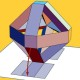#### [SOLVED] How to show the two convex bodies are affinely isomorphic?

This problem comes from the response of the author of papers.

Consider two convex bodies $A$ and $B$:

$$A= \{X\in \mathcal{S}^4 : \operatorname{tr}(X) = 1, X\succeq 0 \}$$
$$B = \operatorname{conv} SO(3)$$

1. $\mathcal{S}^4$ is the set of symmetric $4\times 4$ matrices.
2. $A$ is a $9$ dimensional convex body. ($A$ is symmetric, so $10$ dimensional body, and $\operatorname{tr}(X) = 1$ will decrease one degree of freedom in the diagonal. Just imagine the vectorization of a matrix.) Of course, the extreme points of $A$ are those matrices in $A$ with rank one.
3. $B$ is also a $9$ dimensional convex body (convex hull of rotation matrices).

I have two questions:

1. What is the difference between "affinely isomorphic" and "isomorphic"? I try to search some lectures; however, I cannot fully understand it still. Hope for a plain explanation.
2. How to prove that both convex bodies are affinely isomorphic?#### @Fedor Petrov 2016-09-12 07:37:30

Consider a 3d-rotation with respect to the axis generated by the unit vector $(a,b,c)$ to the angle $\theta$. Its matrix is $$M=\pmatrix{\cos \theta+a^2(1-\cos\theta)&ab(1-\cos\theta)-c\sin\theta& ac(1-\cos\theta)+b\sin\theta \\ab(1-\cos\theta)+c\sin\theta&\cos \theta+b^2(1-\cos\theta)&bc(1-\cos\theta)-a\sin\theta\\ac(1-\cos\theta)-b\sin\theta&bc(1-\cos\theta)+a\sin\theta&\cos \theta+b^2(1-\cos\theta)}.$$ Now take a 4d-projection of rank 1 to the line generated by the unit vector $(a\sin \frac{\theta}2,b\sin \frac{\theta}2,c\sin \frac{\theta}2,\cos \frac{\theta}2)$. Its matrix elements are $\cos^2\frac{\theta}2,a^2\sin^2 \frac{\theta}2$, $ab\sin^2 \frac\theta2$, $a\sin \frac\theta2 \cos \frac\theta2$ and so on. All of them are affine functions of the matrix elements of the above matrix $M$ and viceversa. It follows from the formulae $\cos\theta=2\cos^2\frac{\theta}2-1$, $1-\cos\theta=2\sin^2 \frac\theta2$, $\sin\theta=2\sin \frac\theta2\cos \frac\theta2$. These affine functions define the affine map which maps $A$ onto $B$.#### @sleeve chen 2016-09-12 18:12:20

What does "ace" mean here?#### @Fedor Petrov 2016-09-12 18:23:35

Ah, axis, of course. Invariant line of a rotation.#### @sleeve chen 2016-09-12 20:34:38

Just ask one more trivial question. The affine maps in your answer hold for extreme points in $A$ and $B$. It also holds for other points in $A$ and $B$ just because the affine map preserves convexity?#### @Fedor Petrov 2016-09-12 20:56:55

Yes, of course, affine image of the convex hull of a set is the convex hull of its image.#### @Joseph O'Rourke 2016-09-11 19:37:55

Here is a definition of affinely isomorphic for convex polytopes:Ziegler, Günter M. Lectures on polytopes. Vol. 152. Springer Science & Business Media, 1995. p5.

### [SOLVED] Convex misfit of finite-dimensional convex bodies

• 2018-06-07 05:12:08
• Wlod AA
• 120 View
• 2 Score
• Tags:   convex-geometry

### [SOLVED] An affine characterization of ellipsoids?

• 2017-11-16 16:21:45
• Wlodek Kuperberg
• 237 View
• 8 Score
• Tags:   convex-geometry